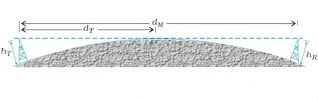# What is the Space Wave Propagation | Range of Transmitting Antenna | Applications

Space wave propagation is the mode of propagation of electromagnetic waves in which a radio wave travels as a space wave in a straight line from...

In the ground wave propagation energy loss increases with increasing in frequency. Therefore, high frequency wave cannot propagate through ground wave propagation, moreover ionosphere is transparent to these very high frequency waves and hence cannot be reflected back from ionosphere. Therefore, Sky wave propagation is not suitable for the propagation of radio waves. Due to this lack, we need to accept space wave propagation for high frequency waves.

## Space Wave Propagation

Space wave propagation is the mode of propagation of electromagnetic waves in which a radio wave travels as a space wave in a straight line from transmitting antenna to the receiving antenna. As space wave travel in straight lines, the propagation is also known as "Line of sight" (LOS) propagation.Space Wave Propagation

In space wave propagation transmitted waves travelling in a straight line directly reach the receiver and are picked up by receiving antenna due to finite curvature of the earth. Such wave cannot be seen beyond the Target Point intercepting the Earth surface. The effective reception range of broad caste is essentially the region between these two points which curved by the line of sight is an conventional sense. Hence, sometimes this mode of communication is called Line of Sight communication.

At frequencies above 40 megahertz (MHz), radio communications is essentially limited to line of sight paths through space waves. TV/RADAR communication, FM radio, microwave links and satellite communications are examples of communication system that use space wave mode of communication.

Different propagation modes for electromagnetic/radio waves are shown in Fig.Different propagation modes

## Range of Transmitting Antenna

In the given figure, ST is the length of transmitting antenna of height (hT) is located at point S on the surface of earth. O is center of the Earth. Due to curvature of the earth, the transmitted signal can be received up to points P and Q which are at maximum distance SP and SQ respectively from the base S of antenna. This points are tangential point on the surface of the earth from tangent TP and TQ draw point T on the curved surface R.

Let SP = SQ = dT i.e. maximum distance upto which transmitted signal can be received.

In ΔOPT, by PGT (Pythagoras Theorem)

OP2 + PT2 = OT2

Re2 + PT2 = (OS+ST)2

Re2 + PT2 = (Re + hT)2

PT2 = hT2 + 2Reh

If h << Re than h2 + 2Re h = 2Reh

PT2 = 2RehT

PT = (2RehT)1/2

dT = (2RehT)1/2

From this formula, it is clear that the radio horizon of the transmitting antenna depends on the height of transmitting antenna only.

## Applications of Space Wave Propagation

Space wave propagation has its application in various system like :

1. Line-of-Sight communication
2. Satellite communication

## Que : The transmitting antenna of Doordarshan Delhi is at the top of a tower of height 252 m. What is range of TV programme telecast by Delhi Doordarshan channel? (Re = 6400 km)

Ans : Here,

hT = 252 m =0.252 km

Re = 6400 km

Therefore, Range of TV channel, dT = (2RehT)1/2

dT = (2×6400×0.252)1/2

dT = (3225.6)1/2

dT = 56.79 km

dT = 57 km